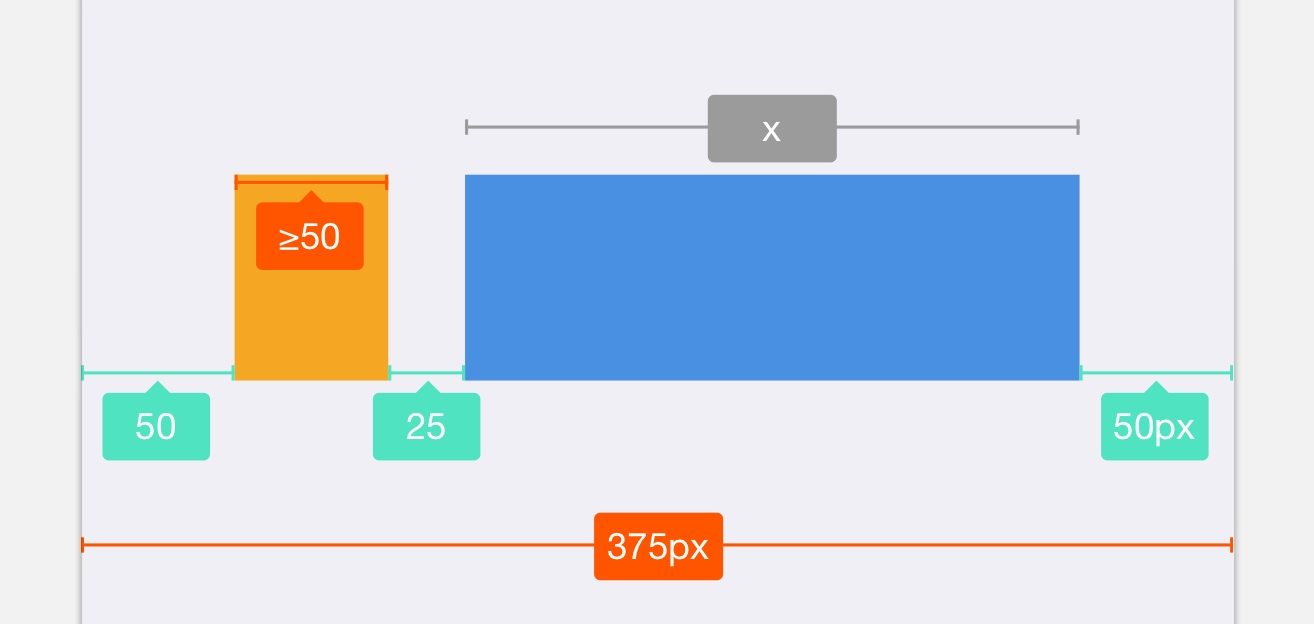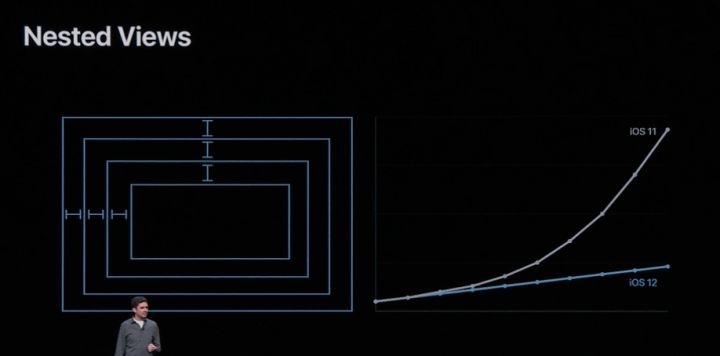# AutoLayout 中的线性规划 - Simplex 算法

## 温习线性规划## Simplex 算法

Simplex 算法主要有三个步骤：

1. 找到一个初始的基本可行解；
2. 持续进行旋转（Pivot）操作；
3. 重复操作 2，直到找到最优解；

Simplex 算法我以下面一个线性规划方程为例：

Degeneracy can prevent the simplex algorithm from terminating, because it can lead to a phenomenon known as cycling: the slack forms at two different iterations of SIMPLEX are identical. Because of degeneracy, SIMPLEX could choose a sequence of pivot operations that leave the objective value unchanged but repeat a slack form within the sequence. Since SIMPLEX is a deterministic algorithm, if it cycles, then it will cycle through the same series of slack forms forever, never terminating.

1. 带入变量 $x_e$：目标条件中，系数为负数的第一个作为带入变量；
2. 替出变量 $x_i$：对所有约束条件中，选择对 $x_e$ 约束强度最大的一式；

## Simplex 算法 Coding 及改善

• 矩阵 A：约束条件的系数矩阵；
• 矩阵 B：约束条件值矩阵；
• 矩阵 C：目标函数系数矩阵；

import numpy as np

class Simplex(object):

def __init__(self, obj, max_mode=False):
# 默认是解决 min LP 问题，如果是最大值用 True，要 mut -1
self.max_mode = max_mode
self.mat = np.array([ + obj]) * (-1 if max_mode else 1)

def add_constraint(self, a, b):
# 增加约束，即在矩阵最后一行增加
self.mat = np.vstack([self.mat, [b] + a])

@property
def solve(self):
# 获得矩阵的纬度
m, n = self.mat.shape
# 零矩阵和对角矩阵上下排列
temp, B = np.vstack([np.zeros((1, m - 1)), np.eye(m - 1)]), list(range(n - 1, n + m - 1))
# 组合系数矩阵
mat = self.mat = np.hstack([self.mat, temp])
# 循环所有目标函数的系数,直到全部没有负数为止
while mat[0, 1:].min() < 0:
# 1. 选择合适的替入和替出变量
# Bland 规则对矩阵做退化操
col = np.where(mat[0, 1:] < 0) + 1
row = np.array([mat[i] / mat[i][col] if mat[i][col] > 0 else 0x7fffffff for i in
range(1, mat.shape)]).argmin() + 1  # find the theta index
# 如果没有替出变量,则说明原问题无界
if mat[row][col] <= 0: return None

# 2. 旋转过程
# 系数归一,整行相除
mat[row] /= mat[row][col]
# 对所有 i!= row 进行 mat[i]= mat[i] - mat[row] * mat[i][col] 操作
ids = np.arange(mat.shape) != row
mat[ids] -= mat[row] * mat[ids, col:col + 1]
B[row] = col
# 返回目标值,若为最小值,则要 * -1,最大值则不用。
# 后面的矩阵是各个解的系数矩阵,基本变量对应 bi,非基本变量为0
# B[i] < n 判断即为删除松弛增加的变量
return mat * (1 if self.max_mode else -1), {B[i]: mat[i, 0] for i in range(1, m) if B[i] < n}

if __name__ == '__main__':
t = Simplex([-1, -14, -6])
t.add_constraint([1, 1, 1], 4)
t.add_constraint([1, 0, 0], 2)
t.add_constraint([0, 0, 1], 3)
t.add_constraint([0, 3, 1], 6)
print(t.solve)
print(t.mat)

'''
(-32.0, {2: 1.0, 3: 3.0})
[[32.   1.   0.   0.   2.   0.   0.   4. ]
[ 1.  -0.5  1.   0.  -0.5  0.   0.   0.5]
[ 3.   1.5  0.   1.   1.5  0.   0.  -0.5]
[ 0.  -1.5  0.   0.  -1.5  0.   1.   0.5]
[ 2.   1.   0.   0.   0.   1.   0.   0. ]]
'''


## 算法再修正

if __name__ == '__main__':
t = Simplex([1, 2])
print(t.solve)
print(t.mat)

'''
(-0.0, {})
[[0. 1. 2. 0. 0.]
[2. 1. 1. 1. 0.]
[1. 1. 1. 0. 1.]]
'''


var x1 >= 0;
var x2 >= 0;

minimize obj: x1 + 2 * x2;

c1: x1 + x2 <= 2;
c2: x1 + x2 >= 1;

solve;
display x1;
display x2;
end;

A: min|aij| =  1.000e+00  max|aij| =  1.000e+00  ratio =  1.000e+00
Problem data seem to be well scaled
Constructing initial basis...
Size of triangular part is 2
0: obj =   0.000000000e+00 inf =   1.000e+00 (1)
1: obj =   1.000000000e+00 inf =   0.000e+00 (0)
OPTIMAL LP SOLUTION FOUND
Time used:   0.0 secs
Memory used: 0.1 Mb (102205 bytes)
Display statement at line 10
x1.val = 1
Display statement at line 11
x2.val = 0
Model has been successfully processed


1. 若 $b_i$ 都为非负数。则直接使用原来的 Simplex 方法进行求解；
2. 否则引入 $x_0$，创建辅助线性规划 $L_aux$；
3. 松弛形式，选择 $b_i$ 最小项的那行带入，$x_0$ 作为替入变量进行一次旋转；
4. 求解 $L_aux$，若最优解为 0，说明有解。否则直接退出；
5. $x_0$ 为基本解，继续旋转，使其成为非基本变量；
6. 恢复原始目标函数，替换变量；
7. 使用原来的 Simplex 求解；
class Simplex(object):

def __init__(self, obj, max_mode=False):
# 默认是解决 min LP 问题，如果是最大值用 True，要 mut -1
self.mat, self.max_mode = np.array([ + obj]) * (-1 if max_mode else 1), max_mode

# 增加约束
def add_constraint(self, a, b):
# 增加约束，即在矩阵最后一行增加
self.mat = np.vstack([self.mat, [b] + a])

# Simplex 算法过程
def _simplex(self, mat, B, m, n):
while mat[0, 1:].min() < 0:
# Bland 规则对矩阵做退化操
col = np.where(mat[0, 1:] < 0) + 1
row = np.array([mat[i] / mat[i][col] if mat[i][col] > 0 else 0x7fffffff for i in
range(1, mat.shape)]).argmin() + 1
# 如果没有替出变量,则说明原问题无界
if mat[row][col] <= 0: return None  # the theta is ∞, the problem is unbounded
self._pivot(mat, B, row, col)
# 返回目标值,若为最小值,则要 * -1,最大值则不用。
# 后面的矩阵是各个解的系数矩阵,基本变量对应 bi,非基本变量为0
# B[i] < n 判断即为删除松弛增加的变量
return mat * (1 if self.max_mode else -1), {B[i]: mat[i, 0] for i in range(1, m) if B[i] < n}

# 旋转过程
def _pivot(self, mat, B, row, col):
# 对所有 i!= row 进行 mat[i]= mat[i] - mat[row] * mat[i][col] 操作
mat[row] /= mat[row][col]
ids = np.arange(mat.shape) != row
mat[ids] -= mat[row] * mat[ids, col:col + 1]
B[row] = col

def solve(self):
# 获得矩阵的纬度
m, n = self.mat.shape
# 组合系数矩阵
temp, B = np.vstack([np.zeros((1, m - 1)), np.eye(m - 1)]), list(range(n - 1, n + m - 1))
# 循环所有目标函数的系数,直到全部没有负数为止
mat = self.mat = np.hstack([self.mat, temp])  # combine them!
# 判断最小的常数 b 是否存在小于 0 的情况,有的话则初始解不可行
if mat[1:, 0].min() < 0:
# 找到最小 b 的那一列
row = mat[1:, 0].argmin() + 1
# temp 保存原先的目标函数, 第0行设置为0
temp, mat = np.copy(mat), 0
# 添加 x0 需要拼接的矩阵,构造辅助线性规划
mat = np.hstack([mat, np.array( + [-1] * (m - 1)).reshape((-1, 1))])
# 执行一次旋转操作,将系数b最小的那一项替出
self._pivot(mat, B, row, mat.shape - 1)
# 求解辅助线性规划,如果最优值为0,则有解,否则无解
if self._simplex(mat, B, m, n) != 0: return None

# 若x0是基本解,需要将 x0 替出
if mat.shape - 1 in B:
# 增加一次旋转来替出 x0
self._pivot(mat, B, B.index(mat.shape - 1), np.where(mat[0, 1:] != 0) + 1)

# 恢复目标函数
self.mat = np.vstack([temp, mat[1:, :-1]])
for i, x in enumerate(B[1:]):
self.mat -= self.mat[0, x] * self.mat[i + 1]
return self._simplex(self.mat, B, m, n)


## 一个简单的场景t = Simplex([0, 1], max_mode=True)
print(t.solve())
print(t.mat)

'''
(200.0, {2: 200.0, 1: 50.0})
[[200.   0.   0.   1.   1.]
[200.   0.   1.   1.   1.]
[ 50.   1.   0.   0.  -1.]]
'''


## 复杂度分析

Simplex 算法再单次约束计算中是否十分高效呢，我们来讨论一下其复杂度。

1. 每次需要查找在目标方程中的一个负数 O(n)
2. 每次需要查找对应项方程 O(m)
3. 旋转操作中，每次需要执行 mat[i] = mat[i] - mat[row] * mat[i][col]。这个操作是对整个行进行操作，并且是按列下标遍历。np.arrange 操作每次会将行列扩展，我们将行定义为最大阈值 m+n，所以旋转开销 O(m*(m+n)) = O(mN)

## 总结